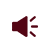• ISSN 1001-1455  CN 51-1148/O3
• EI、Scopus、CA、JST收录
• 力学类中文核心期刊
• 中国科技核心期刊、CSCD统计源期刊引用本文: 曾繁, 冯晓伟, 黄超, 徐权, 肖桂仲, 田荣. 钢筋混凝土结构改进型分离式数值模型[J]. 爆炸与冲击, 2022, 42(6): 065102.ZENG Fan, FENG Xiaowei, HUANG Chao, XU Quan, XIAO Guizhong, TIAN Rong. Modified discrete numerical model for reinforced concrete structures[J]. Explosion And Shock Waves, 2022, 42(6): 065102. doi: 10.11883/bzycj-2021-0286
 Citation: ZENG Fan, FENG Xiaowei, HUANG Chao, XU Quan, XIAO Guizhong, TIAN Rong. Modified discrete numerical model for reinforced concrete structures[J]. Explosion And Shock Waves, 2022, 42(6): 065102.• 中图分类号: O383

## Modified discrete numerical model for reinforced concrete structures

• 摘要: 提出一种考虑粘结滑移效应的钢筋混凝土改进型分离式数值模型。在混合物理论基础上，该模型兼顾混凝土基体和钢筋的力学行为，且基于钢筋混凝土界面粘结滑移模型，获得了钢筋等效模型。改进型分离式数值模型由于对钢筋及其界面无显式离散要求，使得钢筋的运用完全独立于其几何形状，同时对混凝土网格没有约束，并且不增加计算成本，因此该模型可适用于钢筋混凝土宏观结构层面分析。通过钢筋混凝土构件-结构的爆炸实验，对改进型分离式数值模型进行层次化验证。对比结果表明，考虑粘结滑移效应的有限元模型能够更好地预测钢筋混凝土结构的宏观力学行为。
• 图  1  钢筋混凝土框架结构分离式有限元模型

Figure  1.  Improved discrete finite element model of reinforced concrete frame structures

图  2  钢筋尺度模型

Figure  2.  Schematic of the model at steel bar scale

图  3  弹性模量缩放因子$E^{\rm {eq}}_{\rm f}/E_{\rm f}$与粘结区长度l的关系

Figure  3.  Relationship between the scaling factor of the elastic modulus ($E^{\rm {eq}}_{\rm f}/E_{\rm f}$) and the length of the bonding zone (l) under different physical parameters

图  4  塑性硬化模量缩放因子$H^{\rm {eq}}_{\rm f}/H_{\rm f}$与粘结区长度l的关系

Figure  4.  Relationship between the scaling factor of the plastic hardening modulus ($H^{\rm {eq}}_{\rm f}/H_{\rm f}$) and the length of the bonding zone (l) under different physical parameters

图  5  反射超压时程曲线对比

Figure  5.  Comparison of the reflected overpressure-time history curves

图  6  柱竖向位移时程曲线及破坏形貌对比

Figure  6.  Comparison of the vertical displacement-time history curves and failure morphology of columns

图  7  板竖向位移时程曲线对比

Figure  7.  Comparison of the vertical displacement-time history curves of the slab

图  8  网格尺寸对板跨中竖向位移时程曲线的影响

Figure  8.  Effect of mesh size on the vertical displacement-time history curves of the slab

图  9  改进型分离式数值有限元模型及实验（单位：m）

Figure  9.  Improved discrete finite element model and experiment (Unit: m)

图  10  框架结构破坏形貌对比

Figure  10.  Comparison of the failure morphology of the frame structure

图  11  低层中柱位移时程曲线对比

Figure  11.  Comparison of the displacement-time history curves of the lower mid column

表  1  钢筋等效材料参数

Table  1.   Equivalent material parameters of rebar

 钢筋 $f_{\text{y}}^{{\text{eq}}}$/MPa $E_{\text{f}}^{{\text{eq}}}$/GPa $H_{\text{f}}^{{\text{eq}}}$/MPa Vf/% 纵筋 425.0 162.2 788.8 78.5 箍筋 368.1 150.2 656.5 50.0
•点击查看大图
##### 计量
• 文章访问数:  56
• HTML全文浏览量:  118
• PDF下载量:  56
• 被引次数: 0
##### 出版历程
• 收稿日期:  2021-07-05
• 修回日期:  2021-09-17
• 刊出日期:  2022-06-24

### 目录/下载:  全尺寸图片 幻灯片
• 分享
• 用微信扫码二维码

分享至好友和朋友圈